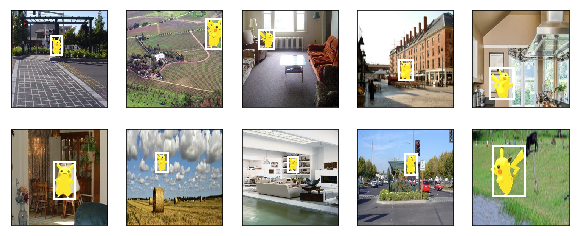# 9.6. 目标检测数据集（皮卡丘）¶

## 9.6.1. 获取数据集¶

RecordIO格式的皮卡丘数据集可以直接在网上下载。获取数据集的操作定义在_download_pikachu函数中。

In :

%matplotlib inline
import d2lzh as d2l
from mxnet import gluon, image
from mxnet.gluon import utils as gutils
import os

root_url = ('https://apache-mxnet.s3-accelerate.amazonaws.com/'
'gluon/dataset/pikachu/')
dataset = {'train.rec': 'e6bcb6ffba1ac04ff8a9b1115e650af56ee969c8',
'train.idx': 'dcf7318b2602c06428b9988470c731621716c393',
'val.rec': 'd6c33f799b4d058e82f2cb5bd9a976f69d72d520'}
for k, v in dataset.items():


## 9.6.2. 读取数据集¶

In :

# 本函数已保存在d2lzh包中方便以后使用
data_dir = '../data/pikachu'
train_iter = image.ImageDetIter(
path_imgrec=os.path.join(data_dir, 'train.rec'),
path_imgidx=os.path.join(data_dir, 'train.idx'),
batch_size=batch_size,
data_shape=(3, edge_size, edge_size),  # 输出图像的形状
shuffle=True,  # 以随机顺序读取数据集
rand_crop=1,  # 随机裁剪的概率为1
min_object_covered=0.95, max_attempts=200)
val_iter = image.ImageDetIter(
path_imgrec=os.path.join(data_dir, 'val.rec'), batch_size=batch_size,
data_shape=(3, edge_size, edge_size), shuffle=False)
return train_iter, val_iter


In :

batch_size, edge_size = 32, 256
batch = train_iter.next()
batch.data.shape, batch.label.shape

Out:

((32, 3, 256, 256), (32, 1, 5))


## 9.6.3. 图示数据¶

In :

imgs = (batch.data[0:10].transpose((0, 2, 3, 1))) / 255
axes = d2l.show_images(imgs, 2, 5).flatten()
for ax, label in zip(axes, batch.label[0:10]):
d2l.show_bboxes(ax, [label[1:5] * edge_size], colors=['w'])## 9.6.4. 小结¶

• 合成的皮卡丘数据集可用于测试目标检测模型。
• 目标检测的数据读取与图像分类的类似。然而，在引入边界框后，标签形状和图像增广（如随机裁剪）发生了变化。

## 9.6.5. 练习¶

• 查阅MXNet文档，image.ImageDetIterimage.CreateDetAugmenter这两个类的构造函数有哪些参数？它们的意义是什么？

## 9.6.6. 参考文献¶

 GluonCV 工具包。https://gluon-cv.mxnet.io/

## 9.6.7. 扫码直达讨论区¶